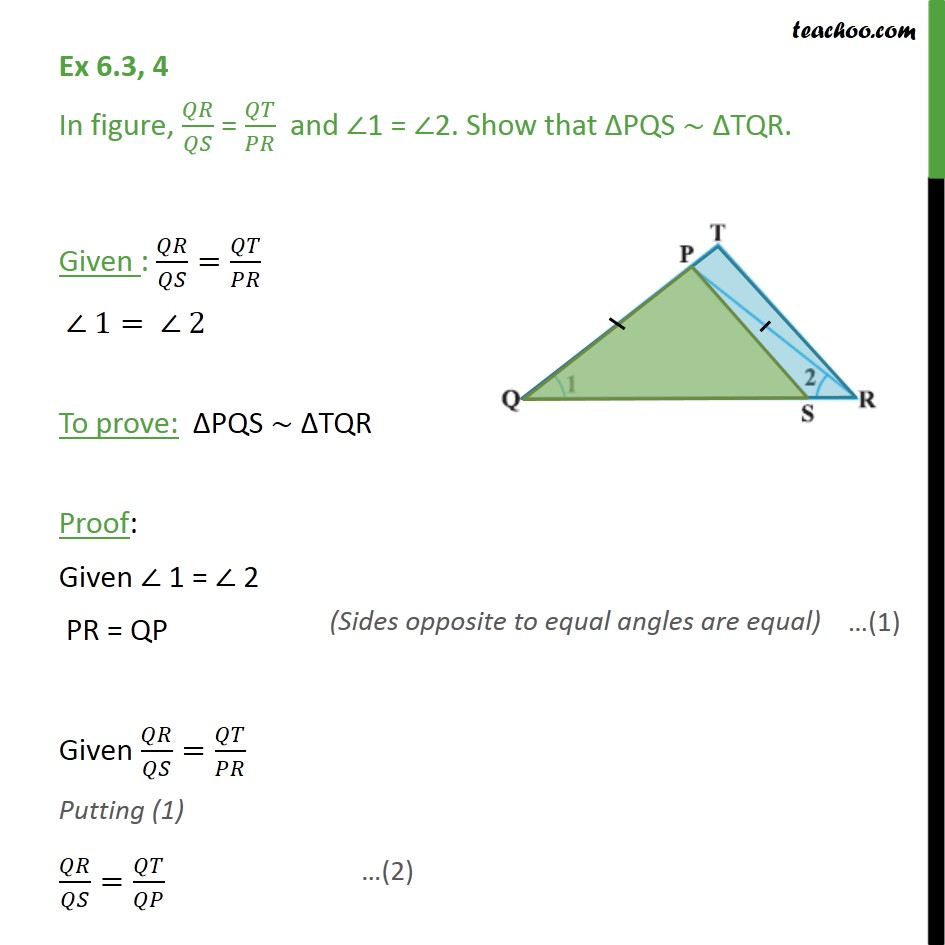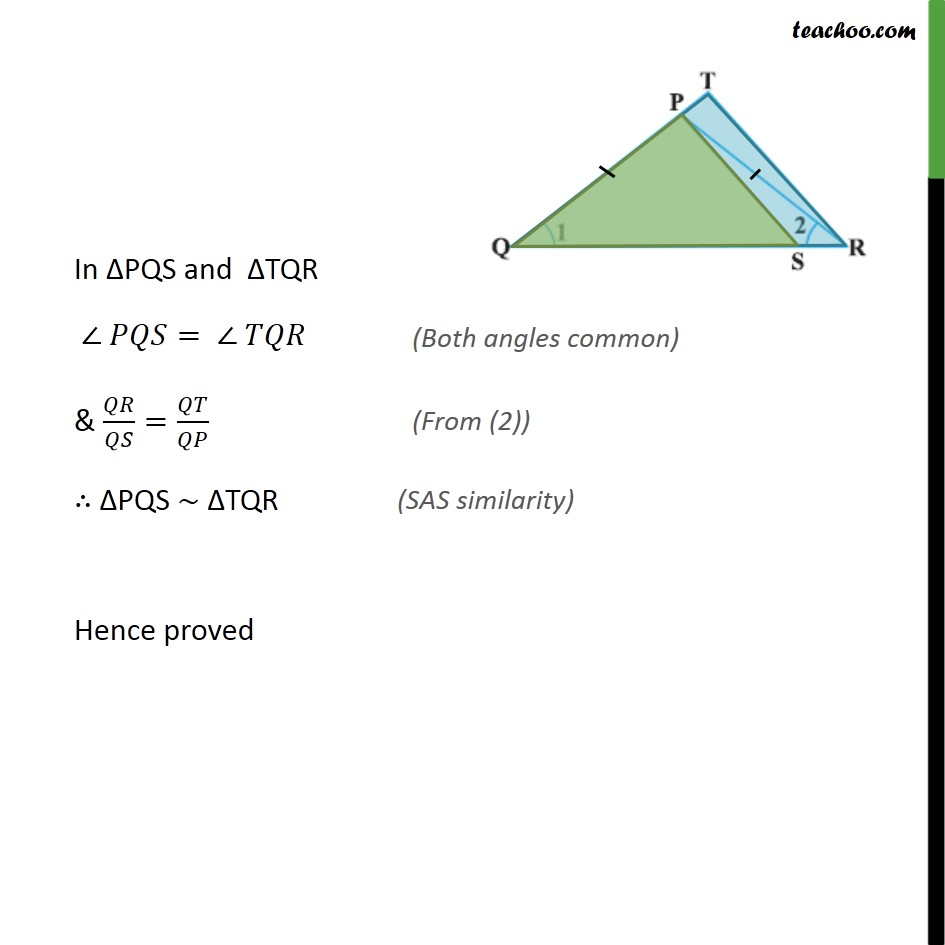1. Chapter 6 Class 10 Triangles
2. Concept wise
3. SAS Similarity

Transcript

Ex 6.3, 4 In figure, / = / and 1 = 2. Show that PQS TQR. Given : / = / 1= 2 To prove: PQS TQR Proof: Given 1 = 2 PR = QP Given / = / Putting (1) / = / In PQS and TQR = & / = / PQS TQR Hence proved

SAS Similarity